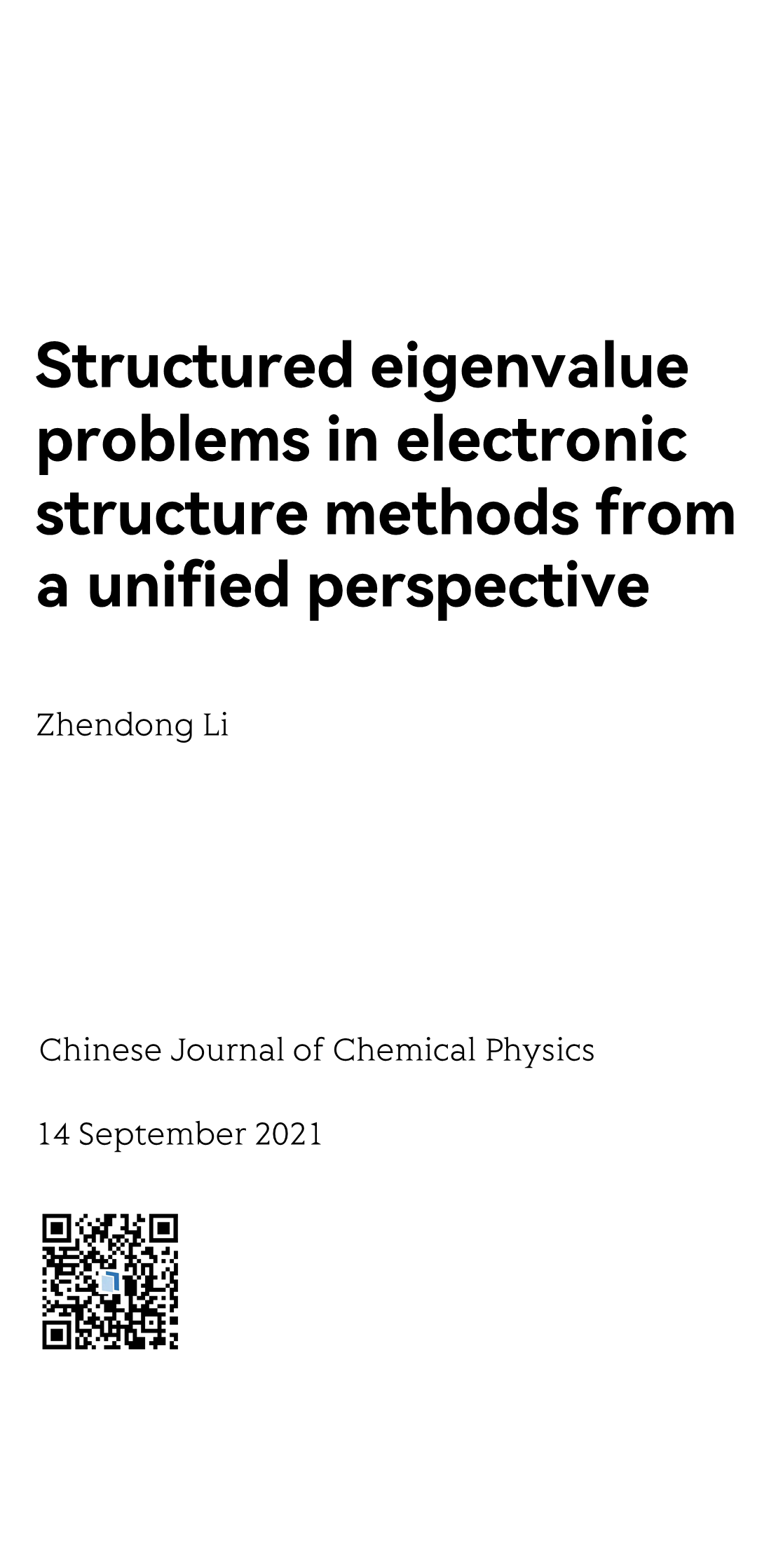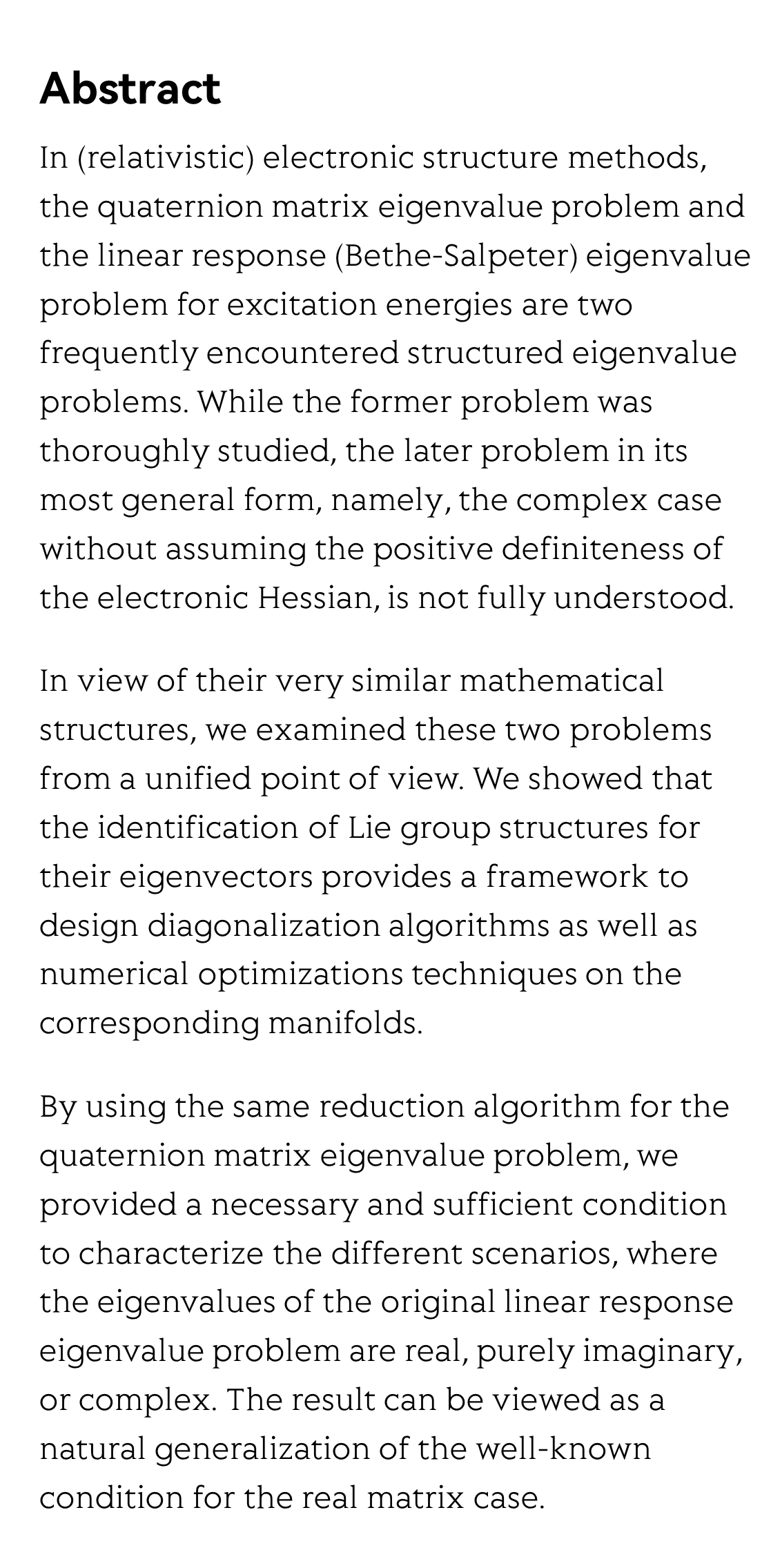MMMYYYYStructured eigenvalue problems in electronic structure methods from a unified perspective

통일된 관점에서 본 전자 구조 방법의 구조적 고유값 문제
Problemas estructurados de valores propios en métodos de estructura electrónica desde una perspectiva unificada
Problèmes de valeurs propres structurés dans les méthodes de structure électronique dans une perspective unifiée
Структурированные проблемы собственных значений в методах электронной структуры с единой точки зрения
Zhendong Li 李振东
Key Laboratory of Theoretical and Computational Photochemistry, Ministry of Education, College of Chemistry, Beijing Normal University, Beijing 100875, China

Chinese Journal of Chemical Physics, 14 September 2021Abstract

In (relativistic) electronic structure methods, the quaternion matrix eigenvalue problem and the linear response (Bethe-Salpeter) eigenvalue problem for excitation energies are two frequently encountered structured eigenvalue problems. While the former problem was thoroughly studied, the later problem in its most general form, namely, the complex case without assuming the positive definiteness of the electronic Hessian, is not fully understood.

In view of their very similar mathematical structures, we examined these two problems from a unified point of view. We showed that the identification of Lie group structures for their eigenvectors provides a framework to design diagonalization algorithms as well as numerical optimizations techniques on the corresponding manifolds.

By using the same reduction algorithm for the quaternion matrix eigenvalue problem, we provided a necessary and sufficient condition to characterize the different scenarios, where the eigenvalues of the original linear response eigenvalue problem are real, purely imaginary, or complex. The result can be viewed as a natural generalization of the well-known condition for the real matrix case.Reviews and Discussions